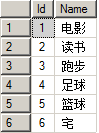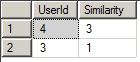# 波斯马BOSSMA Information Technology

## 一个计算相似度的问题

### 示例

1、创建三个表

```CREATE TABLE [dbo].[User](
[Id] [int] IDENTITY(1,1) NOT NULL,
[RealName] [nvarchar](50) NULL,
CONSTRAINT [PK_User] PRIMARY KEY CLUSTERED
(
[Id] ASC
)
) ON [PRIMARY]

CREATE TABLE [dbo].[Interest](
[Id] [int] IDENTITY(1,1) NOT NULL,
[Name] [nvarchar](50) NULL,
CONSTRAINT [PK_Interest] PRIMARY KEY CLUSTERED
(
[Id] ASC
)
) ON [PRIMARY]

CREATE TABLE [dbo].[User2Interest](
[UserId] [int] NOT NULL,
[InterestId] [int] NOT NULL,
CONSTRAINT [PK_User2Interest] PRIMARY KEY CLUSTERED
(
[UserId] ASC,
[InterestId] ASC
)
) ON [PRIMARY]```

2、在数据表中插入一些测试记录：

User：User2Interest：3、查询和某个用户有相同兴趣的其他用户

`select InterestId from User2Interest where UserId = 1`

`select UserId from User2Interest where UserId<>1 and InterestId in (select InterestId from User2Interest where UserId = 1)`

`select UserId,count(1) as similarity from User2Interest where UserId<>1 and InterestId in (select InterestId from User2Interest where UserId = 1) group by UserId order by similarity desc`【上一篇】

### 发表评论